Search by Topic

Resources tagged with Visualising similar to Equal Lengths:

Filter by: Content type:
Age range:
Challenge level:

There are 180 resultsTessellating Hexagons

Age 11 to 14 Challenge Level:

Which hexagons tessellate?All Tied Up

Age 14 to 16 Challenge Level:

A ribbon runs around a box so that it makes a complete loop with two parallel pieces of ribbon on the top. How long will the ribbon be?Tied Up

Age 14 to 16 Short Challenge Level:

How much of the field can the animals graze?Semi-regular Tessellations

Age 11 to 16 Challenge Level:

Semi-regular tessellations combine two or more different regular polygons to fill the plane. Can you find all the semi-regular tessellations?Trice

Age 11 to 14 Challenge Level:

ABCDEFGH is a 3 by 3 by 3 cube. Point P is 1/3 along AB (that is AP : PB = 1 : 2), point Q is 1/3 along GH and point R is 1/3 along ED. What is the area of the triangle PQR?Star Gazing

Age 14 to 16 Challenge Level:

Find the ratio of the outer shaded area to the inner area for a six pointed star and an eight pointed star.Like a Circle in a Spiral

Age 7 to 16 Challenge Level:

A cheap and simple toy with lots of mathematics. Can you interpret the images that are produced? Can you predict the pattern that will be produced using different wheels?Polygon Rings

Age 11 to 14 Challenge Level:

Join pentagons together edge to edge. Will they form a ring?Cutting a Cube

Age 11 to 14 Challenge Level:

A half-cube is cut into two pieces by a plane through the long diagonal and at right angles to it. Can you draw a net of these pieces? Are they identical?Tilting Triangles

Age 14 to 16 Challenge Level:

A right-angled isosceles triangle is rotated about the centre point of a square. What can you say about the area of the part of the square covered by the triangle as it rotates?Triangular Tantaliser

Age 11 to 14 Challenge Level:

Draw all the possible distinct triangles on a 4 x 4 dotty grid. Convince me that you have all possible triangles.Rolling Coins

Age 14 to 16 Challenge Level:

A blue coin rolls round two yellow coins which touch. The coins are the same size. How many revolutions does the blue coin make when it rolls all the way round the yellow coins? Investigate for a. . . .LOGO Challenge - Circles as Animals

Age 11 to 16 Challenge Level:

See if you can anticipate successive 'generations' of the two animals shown here.Three Cubes

Age 14 to 16 Challenge Level:

Can you work out the dimensions of the three cubes?The Old Goats

Age 11 to 14 Challenge Level:

A rectangular field has two posts with a ring on top of each post. There are two quarrelsome goats and plenty of ropes which you can tie to their collars. How can you secure them so they can't. . . .Cube Paths

Age 11 to 14 Challenge Level:

Given a 2 by 2 by 2 skeletal cube with one route `down' the cube. How many routes are there from A to B?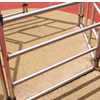Playground Snapshot

Age 7 to 14 Challenge Level:

The image in this problem is part of a piece of equipment found in the playground of a school. How would you describe it to someone over the phone?The Spider and the Fly

Age 14 to 16 Challenge Level:

A spider is sitting in the middle of one of the smallest walls in a room and a fly is resting beside the window. What is the shortest distance the spider would have to crawl to catch the fly?Rolling Around

Age 11 to 14 Challenge Level:

A circle rolls around the outside edge of a square so that its circumference always touches the edge of the square. Can you describe the locus of the centre of the circle?Corridors

Age 14 to 16 Challenge Level:

A 10x10x10 cube is made from 27 2x2 cubes with corridors between them. Find the shortest route from one corner to the opposite corner.Weighty Problem

Age 11 to 14 Challenge Level:

The diagram shows a very heavy kitchen cabinet. It cannot be lifted but it can be pivoted around a corner. The task is to move it, without sliding, in a series of turns about the corners so that it. . . .Triangle Inequality

Age 11 to 14 Challenge Level:

ABC is an equilateral triangle and P is a point in the interior of the triangle. We know that AP = 3cm and BP = 4cm. Prove that CP must be less than 10 cm.Age 14 to 16 Challenge Level:

Four rods are hinged at their ends to form a convex quadrilateral. Investigate the different shapes that the quadrilateral can take. Be patient this problem may be slow to load.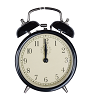On Time

Age 11 to 14 Challenge Level:

On a clock the three hands - the second, minute and hour hands - are on the same axis. How often in a 24 hour day will the second hand be parallel to either of the two other hands?LOGO Challenge - Triangles-squares-stars

Age 11 to 16 Challenge Level:

Can you recreate these designs? What are the basic units? What movement is required between each unit? Some elegant use of procedures will help - variables not essential.Convex Polygons

Age 11 to 14 Challenge Level:

Show that among the interior angles of a convex polygon there cannot be more than three acute angles.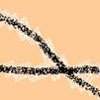Making Tracks

Age 14 to 16 Challenge Level:

A bicycle passes along a path and leaves some tracks. Is it possible to say which track was made by the front wheel and which by the back wheel?Coke Machine

Age 14 to 16 Challenge Level:

The coke machine in college takes 50 pence pieces. It also takes a certain foreign coin of traditional design...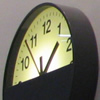Clocking Off

Age 7 to 16 Challenge Level:

I found these clocks in the Arts Centre at the University of Warwick intriguing - do they really need four clocks and what times would be ambiguous with only two or three of them?Efficient Packing

Age 14 to 16 Challenge Level:

How efficiently can you pack together disks?Tetrahedra Tester

Age 11 to 14 Challenge Level:

An irregular tetrahedron is composed of four different triangles. Can such a tetrahedron be constructed where the side lengths are 4, 5, 6, 7, 8 and 9 units of length?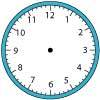Right Time

Age 11 to 14 Challenge Level:

At the time of writing the hour and minute hands of my clock are at right angles. How long will it be before they are at right angles again?Something in Common

Age 14 to 16 Challenge Level:

A square of area 3 square units cannot be drawn on a 2D grid so that each of its vertices have integer coordinates, but can it be drawn on a 3D grid? Investigate squares that can be drawn.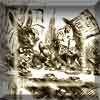A Problem of Time

Age 14 to 16 Challenge Level:

Consider a watch face which has identical hands and identical marks for the hours. It is opposite to a mirror. When is the time as read direct and in the mirror exactly the same between 6 and 7?Age 14 to 16 Challenge Level:

In this problem we are faced with an apparently easy area problem, but it has gone horribly wrong! What happened?Dotty Triangles

Age 11 to 14 Challenge Level:

Imagine an infinitely large sheet of square dotty paper on which you can draw triangles of any size you wish (providing each vertex is on a dot). What areas is it/is it not possible to draw?Getting an Angle

Age 11 to 14 Challenge Level:

How can you make an angle of 60 degrees by folding a sheet of paper twice?Counting Triangles

Age 11 to 14 Challenge Level:

Triangles are formed by joining the vertices of a skeletal cube. How many different types of triangle are there? How many triangles altogether?Efficient Cutting

Age 11 to 14 Challenge Level:

Use a single sheet of A4 paper and make a cylinder having the greatest possible volume. The cylinder must be closed off by a circle at each end.Square It

Age 11 to 16 Challenge Level:

Players take it in turns to choose a dot on the grid. The winner is the first to have four dots that can be joined to form a square.Sliced

Age 14 to 16 Challenge Level:

An irregular tetrahedron has two opposite sides the same length a and the line joining their midpoints is perpendicular to these two edges and is of length b. What is the volume of the tetrahedron?Sliding Puzzle

Age 5 to 16 Challenge Level:

The aim of the game is to slide the green square from the top right hand corner to the bottom left hand corner in the least number of moves.One and Three

Age 14 to 16 Challenge Level:

Two motorboats travelling up and down a lake at constant speeds leave opposite ends A and B at the same instant, passing each other, for the first time 600 metres from A, and on their return, 400. . . .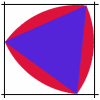Rolling Triangle

Age 11 to 14 Challenge Level:

The triangle ABC is equilateral. The arc AB has centre C, the arc BC has centre A and the arc CA has centre B. Explain how and why this shape can roll along between two parallel tracks.Khun Phaen Escapes to Freedom

Age 11 to 14 Challenge Level:

Slide the pieces to move Khun Phaen past all the guards into the position on the right from which he can escape to freedom.Changing Places

Age 14 to 16 Challenge Level:

Place a red counter in the top left corner of a 4x4 array, which is covered by 14 other smaller counters, leaving a gap in the bottom right hand corner (HOME). What is the smallest number of moves. . . .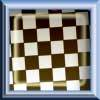Diagonal Dodge

Age 7 to 14 Challenge Level:

A game for 2 players. Can be played online. One player has 1 red counter, the other has 4 blue. The red counter needs to reach the other side, and the blue needs to trap the red.Inside Out

Age 14 to 16 Challenge Level:

There are 27 small cubes in a 3 x 3 x 3 cube, 54 faces being visible at any one time. Is it possible to reorganise these cubes so that by dipping the large cube into a pot of paint three times you. . . .An Unusual Shape

Age 11 to 14 Challenge Level:

Can you maximise the area available to a grazing goat?Picturing Triangular Numbers

Age 11 to 14 Challenge Level:

Triangular numbers can be represented by a triangular array of squares. What do you notice about the sum of identical triangle numbers?